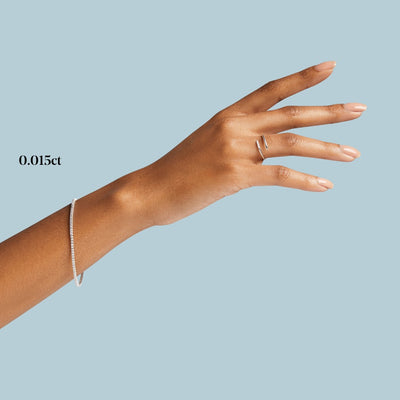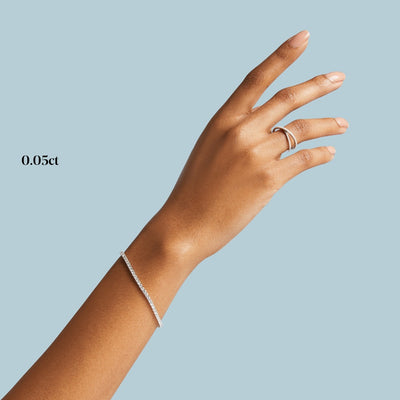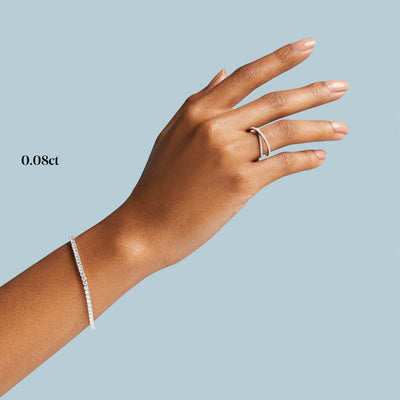# Diamond Tennis Bracelet

Regular price
\$2,150.00
Sale price
2,150.00
Regular price
Sold out
Unit price
per

A classic piece that adds natural sparkle to your life and will never go out of style!

Quantity must be 1 or more

All pieces are custom made in New York City. This piece is available to ship within 2-3 weeks.

- Solid 14k white, yellow, or rose gold

- Featuring natural G-H color, SI clarity round diamonds, 0.015ct - 0.08ct each

- Total carat weight based on 0.015ct: 5.5” = 1.08ctw | 6” = 1.18ctw | 6.5” = 1.28ctw |7” = 1.38ctw | 7.5” = 1.48ctw*

- Total carat weight based on 0.03ct: 5.5” = 1.80ctw |6” = 1.95ctw | 6.5” = 2.10ctw | 7” = 2.25ctw | 7.5” = 2.40ctw*

- Total carat weight based on 0.05ct: 5.5” = 2.65ctw | 6” = 2.90ctw | 6.5” = 3.15ctw |7” = 3.40ctw |7.5” = 3.65ctw*

- Total carat weight based on 0.08ct: 5.5” = 3.88ctw | 6” = 4.24ctw | 6.5” = 4.60ctw | 7” = 4.96ctw | 7.5” = 5.32ctw*

- *Total carat weight will vary based on length

- 1.5" safety chain

- 0.015ct = 2.1mm | 0.03ct = 2.4mm | 0.05ct = 2.6mm | 0.08ct = 2.9mm width

Do you have questions about our products? Check out our FAQs here

• Product details

- Solid 14k white, yellow, or rose gold

- Featuring natural G-H color, SI clarity round diamonds, 0.015ct - 0.08ct each

- Total carat weight based on 0.015ct: 5.5” = 1.08ctw | 6” = 1.18ctw | 6.5” = 1.28ctw |7” = 1.38ctw | 7.5” = 1.48ctw*

- Total carat weight based on 0.03ct: 5.5” = 1.80ctw |6” = 1.95ctw | 6.5” = 2.10ctw | 7” = 2.25ctw | 7.5” = 2.40ctw*

- Total carat weight based on 0.05ct: 5.5” = 2.65ctw | 6” = 2.90ctw | 6.5” = 3.15ctw |7” = 3.40ctw |7.5” = 3.65ctw*

- Total carat weight based on 0.08ct: 5.5” = 3.88ctw | 6” = 4.24ctw | 6.5” = 4.60ctw | 7” = 4.96ctw | 7.5” = 5.32ctw*

- *Total carat weight will vary based on length

- 1.5" safety chain

- 0.015ct = 2.1mm | 0.03ct = 2.4mm | 0.05ct = 2.6mm | 0.08ct = 2.9mm width

• Diamond Details